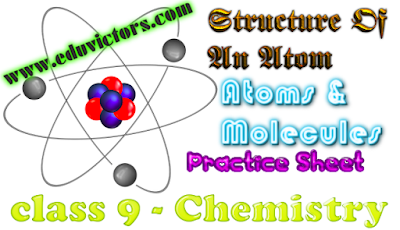## Chemistry - Structure Of An Atom, Atoms & Molecules - Practice Test

M.M = 40

Q1. What are the failures of Dalton Atomic theory? (3)

Q2. State the law of constant Proportion? verify it for the reaction of 2.047g H₂ and 16.166g O₂ giving 18.210g water.(3)

Q3. How many molecules of water are present in a drop of water which has a mass of 50g? (2)

Q4. Oxygen is (a) Monovalent (b) Bivalent (c) Trivalent (d) Tetravalent        (1)

Q5. Define the terms:
a) Atomic number
b) Mass number       (2)

Q6. Which element will be more reactive and why the element whose atomic number is 10 or the one whose atomic number is 11?       (3)

Q7. What are isotopes? Name the isotopes of hydrogen and draw the structure of their atoms? (3)

Q8. Define Valency? Find the Valency of oxygen and Aluminum. (3)

Q9. What are ions? What are its two types? (1)

Q10. Describe Thomson model of an atom.(2)

Q11. Describe Rutherford’s alpha particle scattering experiment. What are its drawbacks? (3+2)

Q12. How Neils Bohr explained the stability of an atom? (2)

Q13. What are the uses of isotopes?  (2)

Q14. How  valency and valence electrons different from each other?  (2)

Q15. Elaborate E.Goldstein’s contribution in providing structure of atom (1)

Q16. The average atomic mass of a sample of an element Y is 16.2u..what are the percentage isotope of its 16u and 18u mass isotope.(3)

Q17. Find the atomic mass of C₆H₁₂O₆ and HNO₃ .(2)